# Python If-Then-Elif-Else Statement

In previous two articles, we discussed conditional statements such as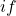and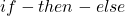. All of those previous structures are using one conditions only, what if, we want to check multiple conditions at different time and make decisions based on the output of those conditions. The python has a special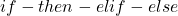construct to check multiple conditions one after another. Look at the figure below to understand this logic better.

Note that the condition 1 comes from if-block, condition 2 comes from elif, condition 3 is elif and else block is D.

The first condition,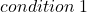is the if-block and if true then executes the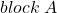and if false will move to the next condition, which is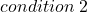. Ifis true, that will execute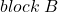and if it is false then move to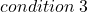. Theif true will execute the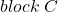and if everything is false, the final output is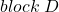.

### Example – If-then-elif-else

In this example, student receive his grade in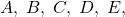and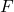.

\begin{aligned}
&A = Excellent\\
&B = Outstanding\\
&C = Very \hspace{3px}Good\\
&D = Good\\
&E = Fair \\
&F = Fail
\end{aligned}

We need to print the result based on the grade that the student gets. Consider the following code.

grade = input("Enter Your Grade:" )
else:
print("Wrong Input")


The output of the program is given below.

### Output – If-then-elif-else

=========== RESTART: C:/Python_projects/Display_Student_Result.py ===========
>>>